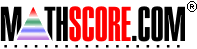Math Practice Online > free > lessons > Florida > 6th grade > Algebraic Word Problems

## Algebraic Word Problems

This topic covers the skill of solving simple algebraic word problems.

 Sample Problems for Algebraic Word Problems Lesson for Algebraic Word Problems

### This topic aligns to the following state standards

Grade 4: Alg 3. Translates problem-solving situations into expressions and equations using a variable for the unknown.
Grade 5: Alg 1. Solves problems involving simple equations or inequalities using diagrams or models, symbolic expressions, or written phrases.
Grade 6: Alg 1. Uses variables to represent numbers and relationships.

Copyright Accurate Learning Systems Corporation 2008.